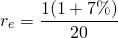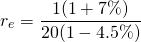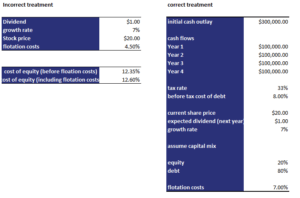# Flotation Costs

Flotation costs are incurred by a company when it raises new capital and are typically between 2% and 6%. We can define flotation costs as the fees charged by investment bankers when a company is raising external capital to finance projects. These flotation costs should be incorporated in the weighted average cost of capital calculation if we want to calculate true cost of capital for projects.

Having discussed the flotation cost definition, we will now discuss two ways in which one can incorporate flotation costs. We also provide an flotation costs example that illustrates the correct way of including these costs. At the bottom of the page, we include a flotation cost calculator in Excel.

## Incorrect treatment flotation costs

How to calculate flotation cost? We mentioned in the introduction that there are two ways of incorporating these costs in the cost of capital. The first approach is considered an incorrect treatment by some, but we also discuss it for completeness.

The first way to consider the costs related to raising equity is by increasing the cost of external equity. For example, consider a company that currently pays a dividend of $1, has a stock price of$20, and has an expected growth rate of 7%. Let’s first calculate the cost of equity without considering the cost of raising equityNext, assume the percentage flotation cost is 4.5%The above flotation cost example increases the cost of capital of equity by xx%.

## Correct treatment

The above flotation cost example increases the cost of equity by a fixed percentage. Using this cost of equity in a weighted average cost of capital (WACC) calculation will mean that flotation costs will be a factor for the duration of the project. This is because all cash flows will be discounted at this higher WACC. Flotation costs, however, are not an ongoing expense. Instead, these costs are a cash outflow at the start of the project. Thus, we should consider the costs as an initial outflow and add it to the initial project cost.

In the Excel spreadsheet at the bottom of the page, we implement the correct flotation cost formula. Here we report the main steps### Flotation cost calculator

Want to calculate flotation costs’ impact on the cost of equity in Excel? Download our free excel file: Flotation costs example